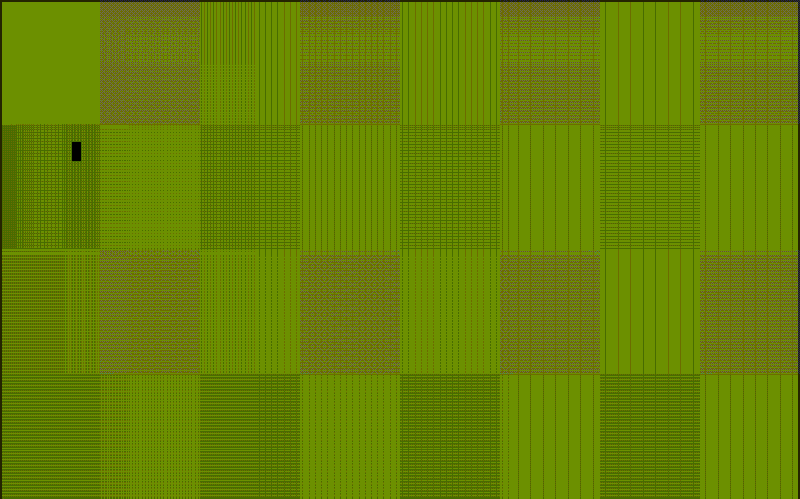# Location¶

If you just want to move the location of the cursor before writing text, and aren’t worried about returning, do something like this:

```>>> print(term.home + term.clear)
>>> print(term.down(2) + term.move_right(20) + term.bright_red('fire!'))
>>> print(term.move_xy(20, 7) + term.bold('Direct hit!'))
>>> print(term.move_y(term.height - 3))
```

There are four direct movement capabilities:

`move_xy(x, y)`
Position cursor at given x, y.
`move_x(x)`
Position cursor at column x.
`move_y(y)`
Position cursor at row y.
`home`
Position cursor at (0, 0).

And four relative capabilities:

`move_up` or `move_up(y)`
Position cursor 1 or y row cells above the current position.
`move_down(y)`

Position cursor 1 or y row cells below the current position.

Note

`move_down` or is often valued as \n, which additionally returns the carriage to
column 0, and, depending on your terminal emulator, may also destroy any characters to end of line.

`move_down(1)` is always a safe non-destructive one-notch movement in the downward direction.

`move_left` or `move_left(x)`
Position cursor 1 or x column cells left of the current position.
`move_right` or `move_right(x)`
Position cursor 1 or x column cells right of the current position.

## Example¶

The source code of bounce.py combines a small bit of Keyboard input with many of the Terminal location capabilities, `home`, `width`, `height`, and `move_xy` are used to create a classic game of tennis:

```# std imports
from math import floor

# local
from blessed import Terminal

def roundxy(x, y):
return int(floor(x)), int(floor(y))

term = Terminal()

x, y, xs, ys = 2, 2, 0.4, 0.3
with term.cbreak(), term.hidden_cursor():
# clear the screen
print(term.home + term.black_on_olivedrab4 + term.clear)

# loop every 20ms
while term.inkey(timeout=0.02) != 'q':
# erase,
txt_erase = term.move_xy(*roundxy(x, y)) + ' '

# bounce,
if x >= (term.width - 1) or x <= 0:
xs *= -1
if y >= term.height or y <= 0:
ys *= -1

# move,
x, y = x + xs, y + ys

# draw !
txt_ball = term.move_xy(*roundxy(x, y)) + '█'
print(txt_erase + txt_ball, end='', flush=True)
```## Context Manager¶

A `contextlib.contextmanager()`, `location()` is provided to move the cursor to an (x, y) screen position and restore the previous position on exit:

```with term.location(0, term.height - 1):
print('Here is the bottom.')

print('This is back where I came from.')
```

All parameters to `location()` are optional, we can use it without any arguments at all to restore the cursor location:

```with term.location():
print(term.move_xy(1, 1) + 'Hi Mom!' + term.clear_eol)
```

Note

calls to `location()` may not be nested.

## Finding The Cursor¶

We can determine the cursor’s current position at anytime using `get_location()`.

This uses a kind of “answer back” sequence that your terminal emulator responds to. Because the terminal may not respond, or may take some time to respond, the `timeout` keyword argument can be specified to return coordinates (-1, -1) after a blocking timeout:

```>>> term.get_location(timeout=5)
(32, 0)
```

The return value of `get_location()` mirrors the arguments of `location()`:

```with term.location(12, 12):
val = term.get_location()
print(val)
```

Produces output, `(12, 12)`

Although this wouldn’t be suggested in most applications because of its latency, it certainly simplifies many applications, and, can also be timed, to make a determination of the round-trip time, perhaps even the bandwidth constraints, of a remote terminal!